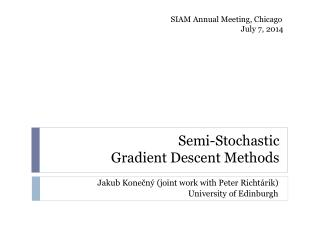Download PresentationDownload Presentation- - - - - - - - - - - - - - - - - - - - - - - - - - - E N D - - - - - - - - - - - - - - - - - - - - - - - - - - -
##### Presentation Transcript

1. SIAM Annual Meeting, Chicago July 7, 2014 Semi-Stochastic Gradient Descent Methods Jakub Konečný(joint work with Peter Richtárik) University of Edinburgh

2. Introduction

3. Large scale problem setting • Problems are often structured • Frequently arising in machine learning is BIG Structure – sum of functions

4. Examples • Linear regression (least squares) • Logistic regression (classification)

5. Assumptions • Lipschitz continuity of derivative of • Strong convexity of

6. Gradient Descent (GD) • Update rule • Fast convergence rate • Alternatively, for accuracy we need iterations • Complexity of single iteration – (measured in gradient evaluations)

7. Stochastic Gradient Descent (SGD) • Update rule • Why it works • Slow convergence • Complexity of single iteration – (measured in gradient evaluations) a step-size parameter

8. Goal GD SGD Fast convergence gradient evaluations in each iteration Slow convergence Complexity of iteration independent of Combine in a single algorithm

10. Intuition • The gradient does not change drastically • We could reuse the information from “old” gradient

11. Modifying “old” gradient • Imagine someone gives us a “good” point and • Gradient at point , near , can be expressed as • Approximation of the gradient Gradient change We can try to estimate Already computed gradient

12. The S2GD Algorithm Simplification; size of the inner loop is random, following a geometric rule

13. Theorem

14. Convergence rate • How to set the parameters ? For any fixed , can be made arbitrarily small by increasing Can be made arbitrarily small, by decreasing

15. Setting the parameters • The accuracy is achieved by setting • Total complexity (in gradient evaluations) Fix target accuracy # of epochs stepsize # of iterations # of epochs cheap iterations full gradient evaluation

16. Complexity • S2GD complexity • GD complexity • iterations • complexity of a single iteration • Total

17. Related Methods • SAG – Stochastic Average Gradient (Mark Schmidt, Nicolas Le Roux, Francis Bach, 2013) • Refresh single stochastic gradient in each iteration • Need to store gradients. • Similar convergence rate • Cumbersome analysis • MISO - Minimization by Incremental Surrogate Optimization (Julien Mairal, 2014) • Similar to SAG, slightly worse performance • Elegant analysis

18. Related Methods • SVRG – Stochastic Variance Reduced Gradient (Rie Johnson, Tong Zhang, 2013) • Arises as a special case in S2GD • Prox-SVRG (Tong Zhang, Lin Xiao, 2014) • Extended to proximal setting • EMGD – Epoch Mixed Gradient Descent (Lijun Zhang, MehrdadMahdavi , Rong Jin, 2013) • Handles simple constraints, • Worse convergence rate

19. Extensions

20. Sparse data • For linear/logistic regression, gradient copies sparsity pattern of example. • But the update direction is fully dense • Can we do something about it? SPARSE DENSE

21. Sparse data • Yes we can! • To compute , we only need coordinates of corresponding to nonzero elements of • For each coordinate , remember when was it updated last time – • Before computing in inner iteration number , update required coordinates • Step being • Compute direction and make a single update The “old gradient” Number of iterations when the coordinate was not updated

22. Sparse data implementation

23. S2GD+ • Observing that SGD can make reasonable progress, while S2GD computes first full gradient (in case we are starting from arbitrary point),we can formulate the following algorithm (S2GD+)

24. S2GD+ Experiment

25. High Probability Result • The result holds only in expectation • Can we say anything about the concentration of the result in practice? For any we have: Paying just logarithm of probabilityIndependent from other parameters

26. Convex loss • Drop strong convexity assumption • Choose start point and define • By running the S2GD algorithm, for any we have,

27. Inexact case • Question: What if we have access to inexact oracle? • Assume we can get the same update direction with error : • S2GD algorithm in this setting gives with

28. Future work • Coordinate version of S2GD • Access to • Inefficient for Linear/Logistic regression, because of “simple” structure • Other problems • Not as simple structure as Linear/Logistic regression • Possibly different computational bottlenecks

29. Code • Efficient implementation for logistic regression -available at MLOSS (soon)